### Greenhouse Effect

The Greenhouse Effect is the process by which the atmosphere of the Earth retains heat which otherwise would be radiated out to space. The name Greenhouse evokes the well known horticultural glass house, however the atmospheric Greenhouse effect has significantly different physical processes and the name often causes misunderstandings. Figure 1 gives a simplified illustration of the radiation exchanges which affect the temperature of the Earth. Radiation from the Sun impacts the Earth and about half hits surface and heats the Earth while different fractions are reflected at the surface and in the atmosphere. The Earth re-radiates part of the heat absorbed and different processes occur which are the greenhouse effect.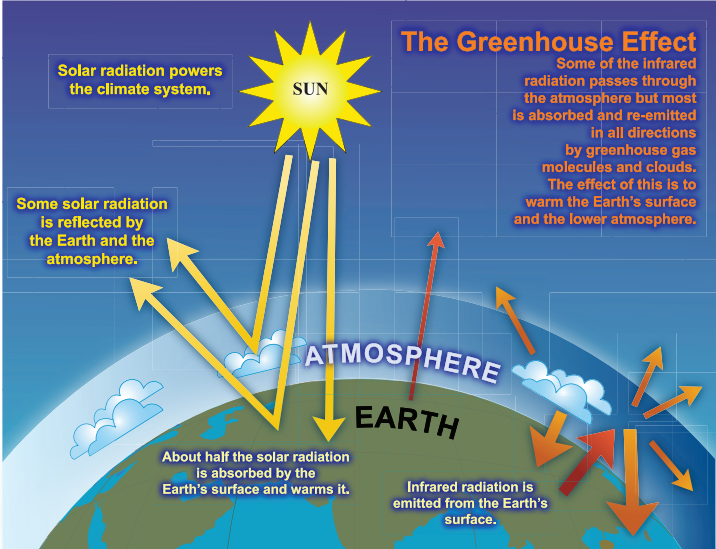Figure 1. An idealised model of the greenhouse effect in the atmosphere of the Earth showing the principle heat exchanges in the atmosphere. The yellow arrows show short wavelength radiation from the Sun and the orange arrows show longwave length radiation emitted from the Earth.

The heat retention happens because the wavelength spectra of radiation from the Sun and from the Earth and the absorption in the atmosphere is different for the two ranges of wavelength. Figure 2 shows these two radiation spectra for Sun and Earth which both follow a Planck black-body distribution but with different average wavelengths because of the average temperatures of the two sources. Figure 3 shows how these ranges of wavelength are absorbed in the atmosphere.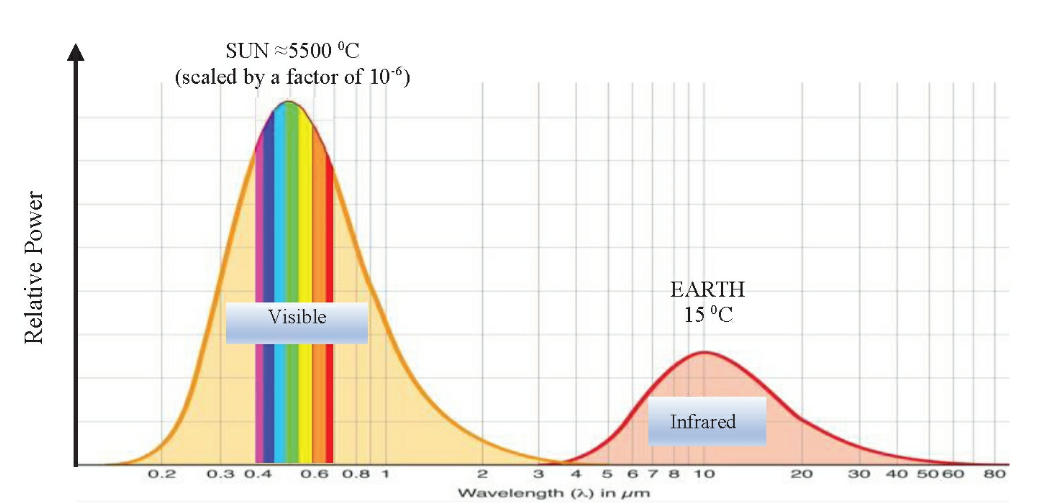Figure 2. Planck black-body emission spectrum for the Sun and Earth, as a function of wavelength. The Sun radiates at visible wavelengths (short wavelengths: SW) because of the high surface temperature of 5500° C and the Earth at infrared wavelengths (long wavelengths: LW) for a surface temperature of 15 °C.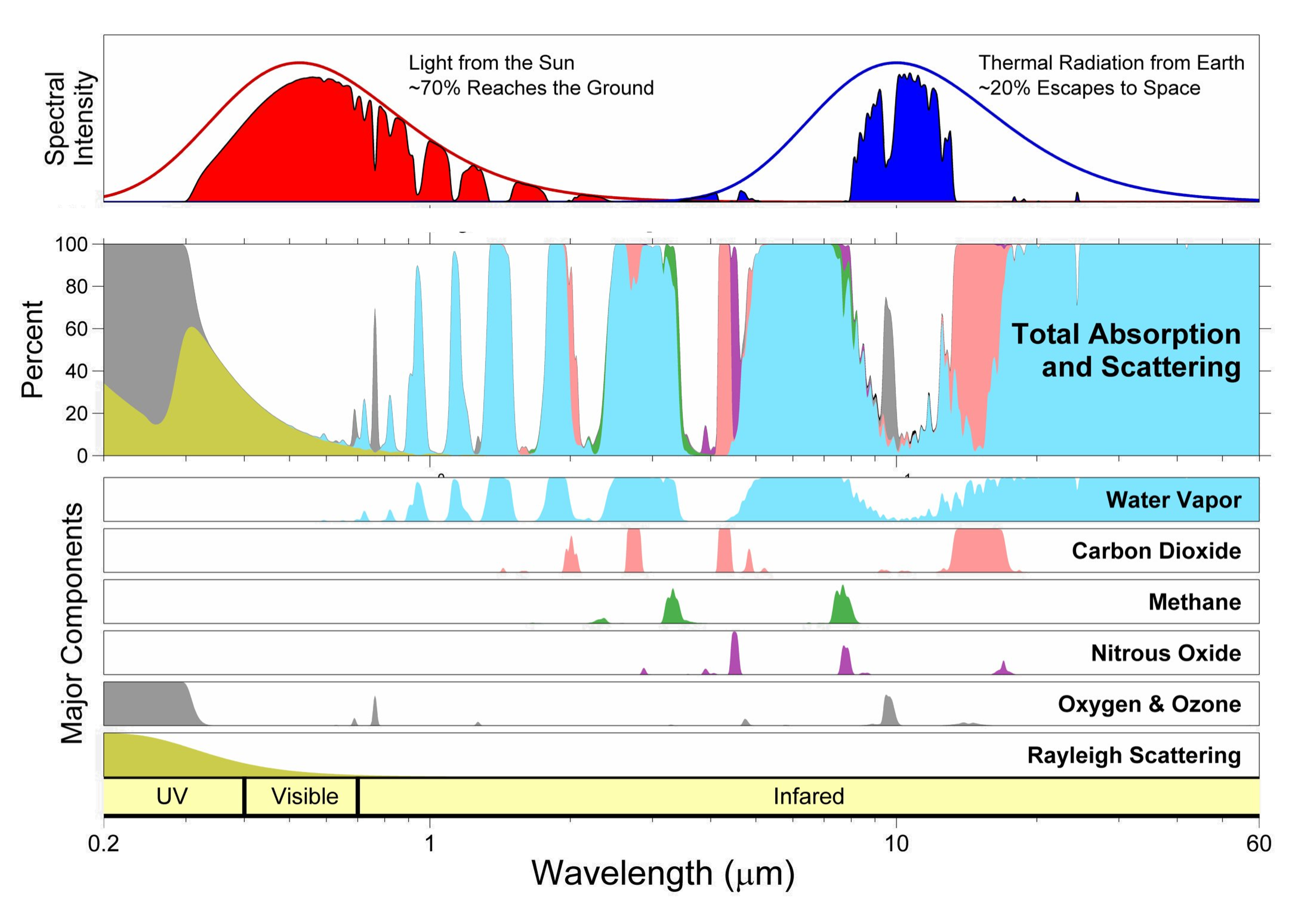Figure 3. Radiation transmission in the atmosphere for wavelengths between 0.2 and 60 micrometres. The bottom plots show the absorption of the trace gases in the atmosphere, with water vapour in blue, carbon dioxides in pink; methane in green; nitrous oxide in purple and oxygen and ozone in grey. The green area in the ultraviolet range show the losses due to Rayleigh scattering. The middle plot combines the individual contributions to give the total absorption and scattering bands. The top plot indicates the fraction of the black-body spectrum of Sun (red) and Earth (blue) which is transmitted with the various losses.

The top plot of figure 3 shows that about 70% of the radiation from the Sun arrives at the surface of the Earth and that about 30% of the radiation re-radiated from the Earth escape to space.

The details of how the greenhouse effect work in the atmosphere are complex. The explanation in this section is taken from the book by Eli Tziperman, Global Warming Science: A Quantitative Introduction to Climate Change and Its Consequences.

The cartoons in figure 2 illustrate the Greenhouse Effect. The first figure1(a) shows an Earth without an atmosphere where shortwave radiation from the sun arrives at the Earth with a fraction being reflected and a fraction being absorbed, together with an amount of longwave radiation being radiated from the surface.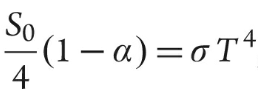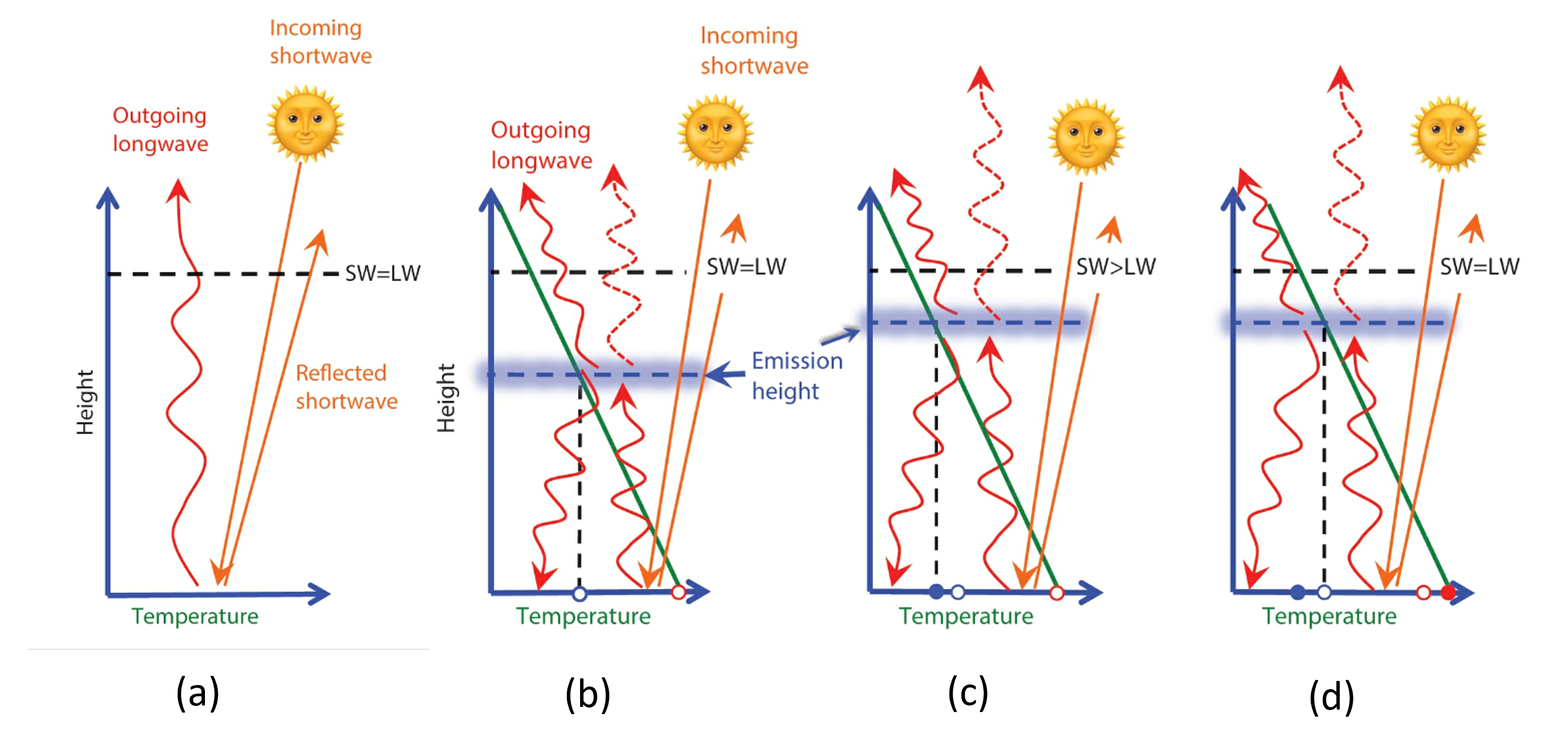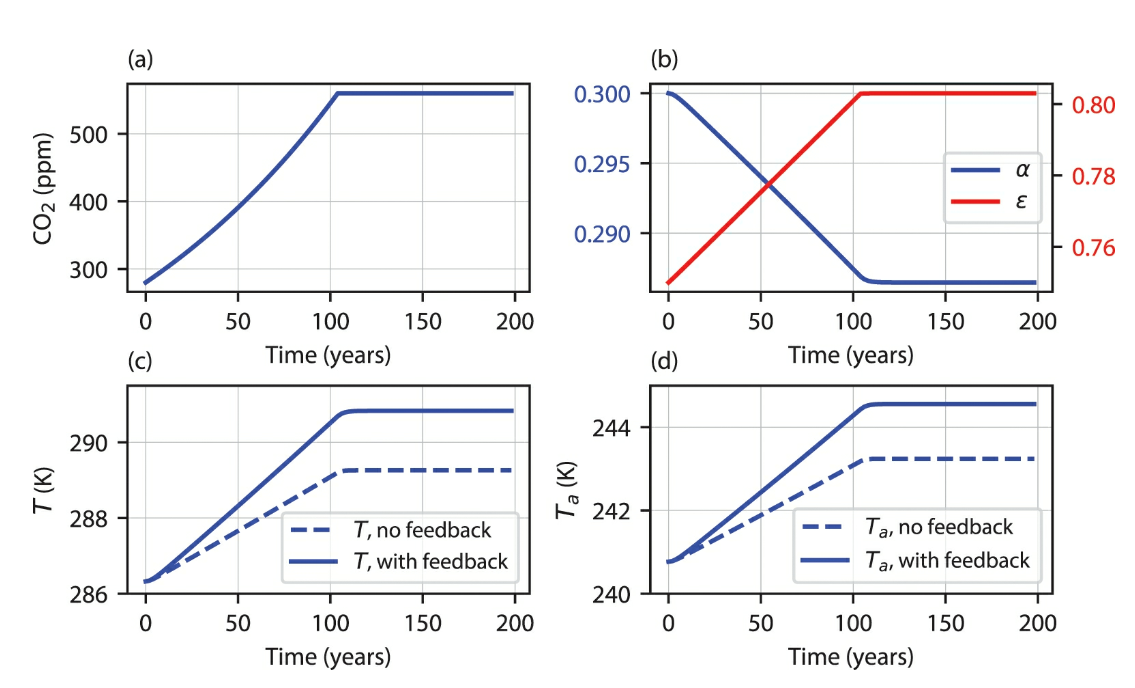Figure 2. Cloud feedbacks in a two-layer energy balance model. Response of a two-level energy balance model to SW and LW cloud feedbacks. (a) Atmospheric CO2 as a function of time, representing a doubling scenario. (b) The change to cloud albedo and emissivity resulting from the formulation in eqn (7.3). (c) The surface temperature as a function of time with and without cloud feedbacks. (d) Same, for the atmospheric temperature.